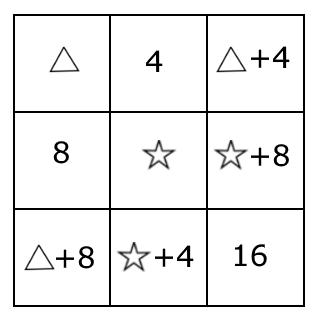#### You may also like### Always Two

Find all the triples of numbers a, b, c such that each one of them plus the product of the other two is always 2.### Not Continued Fractions

Which rational numbers cannot be written in the form x + 1/(y + 1/z) where x, y and z are integers?### Surds

Find the exact values of x, y and a satisfying the following system of equations: 1/(a+1) = a - 1 x + y = 2a x = ay

# Table Total

##### Age 14 to 16 Short Challenge Level:In each row of the table, the third cell contains the sum of the contents of the first two cells.

In each column of the table, the third cell contains the sum of the contents of the first two cells.

What total would you get if you added up the contents of all nine cells?

This problem is adapted from the World Mathematics Championships

You can find more short problems, arranged by curriculum topic, in our short problems collection.